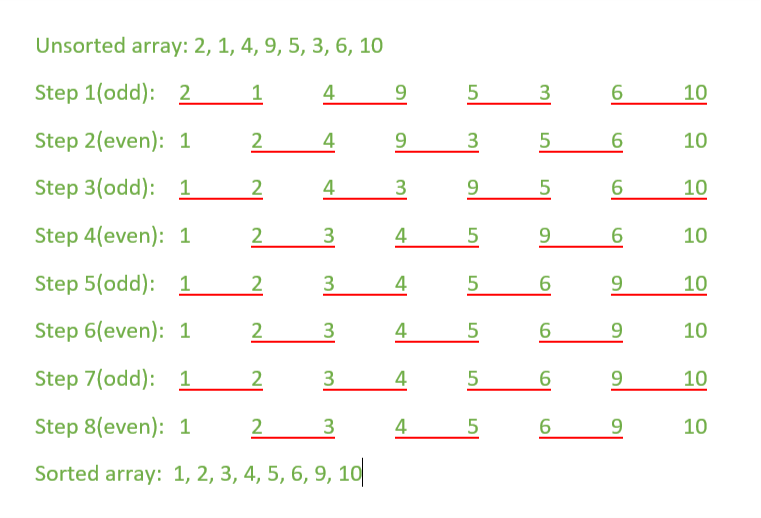GFG App
Open AppBrowser
Continue

# Odd Even Transposition Sort / Brick Sort using pthreads

Odd-Even Transposition Sort is a parallel sorting algorithm. It is based on the Bubble Sort technique, which compares every 2 consecutive numbers in the array and swap them if first is greater than the second to get an ascending order array. It consists of 2 phases – the odd phase and even phase:

• Odd phase: Every odd indexed element is compared with the next even indexed element(considering 1-based indexing).
• Even phase: Every even indexed element is compared with the next odd indexed element.

This article uses the concept of multi-threading, specifically pthread. In each iteration, every pair of 2 consecutive elements is compared using individual threads executing in parallel as illustrated below.Examples:

```Input: { 2, 1, 4, 9, 5, 3, 6, 10 }
Output: 1, 2, 3, 4, 5, 6, 9, 10

Input: { 11, 19, 4, 20, 1, 22, 25, 8}
Output: 1, 4, 8, 11, 19, 20, 22, 25```

Note: Compile the program using following command on your Linux based system.

`g++ program_name.cpp -pthread`

Below is the implementation of the above topic:

## CPP

 `// CPP Program for Odd-Even Transposition sort` `// using pthreads`   `#include ` `#include `   `using` `namespace` `std;`   `// size of array` `#define n 8`   `// maximum number of threads` `int` `max_threads = (n + 1) / 2;`   `int` `a[] = { 2, 1, 4, 9, 5, 3, 6, 10 };` `int` `tmp;`   `// Function to compare and exchange` `// the consecutive elements of the array` `void``* compare(``void``* arg)` `{`   `    ``// Each thread compares` `    ``// two consecutive elements of the array` `    ``int` `index = tmp;` `    ``tmp = tmp + 2;`   `    ``if` `((index + 1 < n) && (a[index] > a[index + 1])) {` `        ``swap(a[index], a[index + 1]);` `    ``}` `}`   `void` `oddEven(pthread_t threads[])` `{` `    ``int` `i, j;`   `    ``for` `(i = 1; i <= n; i++) {` `        ``// Odd step` `        ``if` `(i % 2 == 1) {` `            ``tmp = 0;`   `            ``// Creating threads` `            ``for` `(j = 0; j < max_threads; j++)` `                ``pthread_create(&threads[j], NULL, compare, NULL);`   `            ``// joining threads i.e. waiting` `            ``// for all the threads to complete` `            ``for` `(j = 0; j < max_threads; j++)` `                ``pthread_join(threads[j], NULL);` `        ``}`   `        ``// Even step` `        ``else` `{` `            ``tmp = 1;`   `            ``// Creating threads` `            ``for` `(j = 0; j < max_threads - 1; j++)` `                ``pthread_create(&threads[j], NULL, compare, NULL);`   `            ``// joining threads i.e. waiting` `            ``// for all the threads to complete` `            ``for` `(j = 0; j < max_threads - 1; j++)` `                ``pthread_join(threads[j], NULL);` `        ``}` `    ``}` `}`   `// Function to print an array` `void` `printArray()` `{` `    ``int` `i;` `    ``for` `(i = 0; i < n; i++)` `        ``cout << a[i] << ``" "``;` `    ``cout << endl;` `}`   `// Driver Code` `int` `main()` `{`   `    ``pthread_t threads[max_threads];`   `    ``cout << ``"Given array is: "``;` `    ``printArray();`   `    ``oddEven(threads);`   `    ``cout << ``"\nSorted array is: "``;` `    ``printArray();`   `    ``return` `0;` `}`

## Python3

 `# Python3 Program for Odd-Even Transposition sort` `# using pthreads` `from` `threading ``import` `Thread`   `# Size of array` `N ``=` `8` `# maximum number of threads` `MAX_THREAD ``=` `int``((N``+``1``)``/``2``)` `arr ``=` `[``2``, ``1``, ``4``, ``9``, ``5``, ``3``, ``6``, ``10``]` `tmp ``=` `0`   `# Function to compare and exchange` `# the consecutive elements of the array` `def` `compare():` `    ``global` `tmp` `    ``# Each thread compares` `    ``# two consecutive elements of the array` `    ``index ``=` `tmp` `    ``tmp ``=` `tmp ``+` `2` `    ``if` `index``+``1` `< N ``and` `arr[index] > arr[index``+``1``]:` `        ``arr[index], arr[index``+``1``] ``=` `arr[index``+``1``], arr[index]`     `def` `createThreads():` `    ``# creating list of size MAX_THREAD` `    ``threads ``=` `list``(``range``(MAX_THREAD))` `    `  `    ``# creating MAX_THEAD number of threads` `    ``for` `index ``in` `range``(MAX_THREAD):` `        ``threads[index] ``=` `Thread(target``=``compare)` `        ``threads[index].start()` `        `  `    ``# Waiting for all threads to finish` `    ``for` `index ``in` `range``(MAX_THREAD):` `        ``threads[index].join()`     `def` `oddEven():` `    ``global` `tmp` `    ``for` `i ``in` `range``(``1``, N``+``1``):` `        ``# Odd Step` `        ``if` `i ``%` `2``:` `            ``tmp ``=` `0` `            ``createThreads()` `        ``# Even Step` `        ``else``:` `            ``tmp ``=` `1` `            ``createThreads()`   `# Driver Code` `if` `__name__ ``=``=` `"__main__"``:` `    ``print``(``"Given array is : %s"` `%` `arr)` `    ``oddEven()` `    ``print``(``"Sorted array is : %s"` `%` `arr)`

Output:

```Given array is:  2 1 4 9 5 3 6 10
Sorted array is: 1 2 3 4 5 6 9 10```

Time complexity: The time complexity is reduced to O(N) due to parallel computation using threads. Work complexity: The work complexity of this program is O(N) as N/2 number of threads(resources) are being used to sort the array. So, the work-time complexity of the program is O(N^2).

My Personal Notes arrow_drop_up